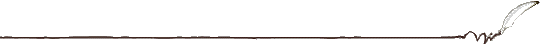## The Quantum Information Manifold for e-bounded Forms

by M. R. Grasselli and R. F. Streater, Reports on Mathmatical Physics, 46, No. 3, 325-335, 2000.

### Abstract

Let H be a self-adjoint operator greater than I and let V be a form-small perturbation such that the operator-norm of $R^{1/2+\epsilon}VR^{1/2-\epsilon}$ is finite. Here, epsilon lies in (0,1) and R is the inverse of H. Suppose that there exists a positive beta less than 1, such that Z, the trace of exp(-beta H), is finite. Let Z(V) be the trace of exp(-beta(H+V)). Then we show that the free energy psi:=log Z is a real analytic function of V in the sense of Fréchet, and that the family of density operators defined in this way is an analytic manifold.